# Tamil Nadu Board Samacheer Kalvi solutions for Class 4th Mathematics Term 3 Answers Guide chapter 2 - Numbers [Latest edition]

#### Chapters## Chapter 2: Numbers

Exercise 2.1Exercise 2.2Exercise 2.3Exercise 2.4Exercise 2.5Exercise 2.6Exercise 2.7
Exercise 2.1 [Page 8]

### Tamil Nadu Board Samacheer Kalvi solutions for Class 4th Mathematics Term 3 Answers Guide Chapter 2 NumbersExercise 2.1 [Page 8]

Exercise 2.1 | Q 1 | Page 8

Simplify the following

896 ÷ 5

Exercise 2.1 | Q 2 | Page 8

Simplify the following

696 ÷ 6

Exercise 2.1 | Q 3 | Page 8

Simplify the following

686 ÷ 7

Exercise 2.1 | Q 4 | Page 8

Simplify the following

813 ÷ 8

Exercise 2.1 | Q 5 | Page 8

Simplify the following

891 ÷ 8

Exercise 2.1 | Q 6 | Page 8

Simplify the following

703 ÷ 2

Exercise 2.1 | Q 7 | Page 8

Simplify the following

Rahul has 192 toy cars. He put them equally in 6 boxes. How many toy cars will he put in each box? How many toy cars are left over? 8) Akila has 4

Exercise 2.1 | Q 8 | Page 8

Simplify the following

Akila has 495 photographs to put in an album. She can put 9 photographs on each page. How many pages can she fill?

Exercise 2.2 [Page 9]

### Tamil Nadu Board Samacheer Kalvi solutions for Class 4th Mathematics Term 3 Answers Guide Chapter 2 NumbersExercise 2.2 [Page 9]

#### Divide the following

Exercise 2.2 | Q 1 | Page 9

5632 ÷ 6

Exercise 2.2 | Q 2 | Page 9

7460 ÷ 7

Exercise 2.2 | Q 3 | Page 9

4964 ÷ 8

Exercise 2.2 | Q 4 | Page 9

8616 ÷ 6

Exercise 2.2 | Q 5 | Page 9

8645 ÷ 7

Exercise 2.2 | Q 6 | Page 9

5742 ÷ 9

Exercise 2.2 | Q 7 | Page 9

In my school, there are 1115 students from class 1 to 8. If the number of students is same in all the classes, how many students are there in each class?

Exercise 2.2 | Q 8 | Page 9

The height of a mountain is 7821 m. Raj took 9 days to reach the top. How many meters did he cover daily if he travelled equal distances every day?

Exercise 2.2 | Q 9 | Page 9

A total of 1787 kg wheat has to be packed equally in 7 bags. What will be the weight of each bag?

Exercise 2.3 [Page 11]

### Tamil Nadu Board Samacheer Kalvi solutions for Class 4th Mathematics Term 3 Answers Guide Chapter 2 NumbersExercise 2.3 [Page 11]

Exercise 2.3 | Page 11
 S.No. Name of the Item Price Per Kg (in ₹) 1 Raw Rice 56 2 Boiled Rice 54 3 Wheat 40 4 Tamarind 180 5 Red chilly 100

What else could you frame some more questions based on this table?
________________________________________

________________________________________

________________________________________

________________________________________

Exercise 2.3 | Q 1 | Page 11

Frame word problems, using the picture given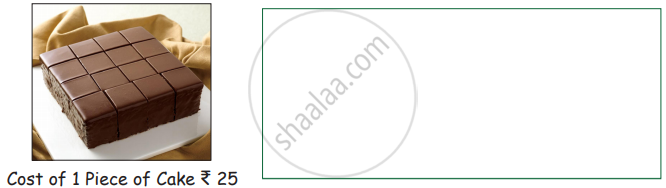Exercise 2.3 | Q 2 | Page 11

Frame word problems, using the picture given: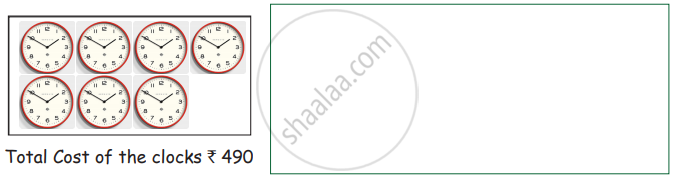Exercise 2.4 [Page 12]

### Tamil Nadu Board Samacheer Kalvi solutions for Class 4th Mathematics Term 3 Answers Guide Chapter 2 NumbersExercise 2.4 [Page 12]

Exercise 2.4 | Q 1 | Page 12

Estimate the following numbers to nearest tens and then add or subtract.Exercise 2.4 | Q 2 | Page 12

Estimate the following numbers to nearest tens and then add or subtract.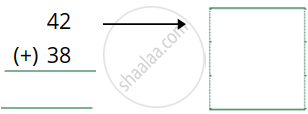Exercise 2.4 | Q 3 | Page 12

Estimate the following numbers to nearest tens and then add or subtract.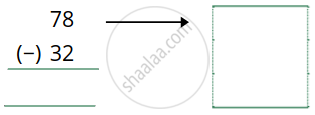Exercise 2.4 | Q 4 | Page 12

Estimate the following numbers to nearest tens and then add or subtract.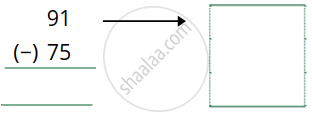Exercise 2.5 [Page 13]

### Tamil Nadu Board Samacheer Kalvi solutions for Class 4th Mathematics Term 3 Answers Guide Chapter 2 NumbersExercise 2.5 [Page 13]

Exercise 2.5 | Q 1 | Page 13

Estimate and calculate:

 S.No. Multiplication fact Actual value Estimated value Difference 1. 35 × 12 420 40 × 10 = 400 20 2. 82 × 28 3. 16 × 12 4. 23 × 27
Exercise 2.6 [Page 16]

### Tamil Nadu Board Samacheer Kalvi solutions for Class 4th Mathematics Term 3 Answers Guide Chapter 2 NumbersExercise 2.6 [Page 16]

Exercise 2.6 | Q 1 | Page 16

Add and subtract the following problem using multiples of 10, 100 (Mentally)

745 + 40 = ___________

Exercise 2.6 | Q 2 | Page 16

Add and subtract the following problem using multiples of 10, 100 (Mentally)

328 + 30 = _________

Exercise 2.6 | Q 3 | Page 16

Add and subtract the following problem using multiples of 10, 100 (Mentally)

566 + 20 = _________

Exercise 2.6 | Q 4 | Page 16

Add and subtract the following problem using multiples of 10, 100 (Mentally)

457 + 100 = _________

Exercise 2.6 | Q 5 | Page 16

Add and subtract the following problem using multiples of 10, 100 (Mentally)

686 + 300 = _________

Exercise 2.6 | Q 6 | Page 16

Add and subtract the following problem using multiples of 10, 100 (Mentally)

345 + 600 = _________

Exercise 2.6 | Q 7 | Page 16

Add and subtract the following problem using multiples of 10, 100 (Mentally)

6348 − 10 = _________

Exercise 2.6 | Q 8 | Page 16

Add and subtract the following problem using multiples of 10, 100 (Mentally)

541 − 40 = _________

Exercise 2.6 | Q 9 | Page 16

Add and subtract the following problem using multiples of 10, 100 (Mentally)

495 − 300 = _________

Exercise 2.6 | Q 10 | Page 16

Add and subtract the following problem using multiples of 10, 100 (Mentally)

657 − 500 = _________

Exercise 2.6 | Q 11 | Page 16

Add and subtract the following problem using multiples of 10, 100 (Mentally)

895 − 500 = _________

Exercise 2.6 | Q 12 | Page 16

Add and subtract the following problem using multiples of 10, 100 (Mentally)

365 − 300 = _________

Exercise 2.7 [Page 17]

### Tamil Nadu Board Samacheer Kalvi solutions for Class 4th Mathematics Term 3 Answers Guide Chapter 2 NumbersExercise 2.7 [Page 17]

Exercise 2.7 | Q 1 | Page 17

Simplify the following multiplication fact by adding partial product.

9 × 42

Exercise 2.7 | Q 2 | Page 17

Simplify the following multiplication fact by adding partial product.

3 × 78

Exercise 2.7 | Q 3 | Page 17

Simplify the following multiplication fact by adding partial product.

36 × 12

Exercise 2.7 | Q 4 | Page 17

Simplify the following multiplication fact by adding partial product.

18 × 19

Exercise 2.7 | Q 5 | Page 17

Simplify the following multiplication fact by adding partial product.

68 × 31

Exercise 2.7 | Q 6 | Page 17

Simplify the following multiplication fact by adding partial product.

42 × 21

## Chapter 2: Numbers

Exercise 2.1Exercise 2.2Exercise 2.3Exercise 2.4Exercise 2.5Exercise 2.6Exercise 2.7## Tamil Nadu Board Samacheer Kalvi solutions for Class 4th Mathematics Term 3 Answers Guide chapter 2 - Numbers

Tamil Nadu Board Samacheer Kalvi solutions for Class 4th Mathematics Term 3 Answers Guide chapter 2 (Numbers) include all questions with solution and detail explanation. This will clear students doubts about any question and improve application skills while preparing for board exams. The detailed, step-by-step solutions will help you understand the concepts better and clear your confusions, if any. Shaalaa.com has the Tamil Nadu Board of Secondary Education Class 4th Mathematics Term 3 Answers Guide solutions in a manner that help students grasp basic concepts better and faster.

Further, we at Shaalaa.com provide such solutions so that students can prepare for written exams. Tamil Nadu Board Samacheer Kalvi textbook solutions can be a core help for self-study and acts as a perfect self-help guidance for students.

Concepts covered in Class 4th Mathematics Term 3 Answers Guide chapter 2 Numbers are Division: (Up to 4 Digit Number by Single Digit Number), To Estimate Sum Or Difference, Estimating Products of Numbers, Mental Arithmetic.

Using Tamil Nadu Board Samacheer Kalvi Class 4th solutions Numbers exercise by students are an easy way to prepare for the exams, as they involve solutions arranged chapter-wise also page wise. The questions involved in Tamil Nadu Board Samacheer Kalvi Solutions are important questions that can be asked in the final exam. Maximum students of Tamil Nadu Board of Secondary Education Class 4th prefer Tamil Nadu Board Samacheer Kalvi Textbook Solutions to score more in exam.

Get the free view of chapter 2 Numbers Class 4th extra questions for Class 4th Mathematics Term 3 Answers Guide and can use Shaalaa.com to keep it handy for your exam preparation